# The Difference Between Beta And Delta And Why We Care

Mar. 13, 2016 1:09 AM ET5 Comments

Beta is one of the classic measurements within the financial industry. It is one of the first measurements shown on Yahoo Finance, right under the bid-ask and earnings estimate. Participants use it as a general gauge of market-related risk associated with an investment. As a reminder, an investment with a Beta of 0.8 is generally supposed to participate in 80% of a market move. So if the market increases 10% the investment should move up 8% and in a down 10% environment it should move down 8%. If only it was so simple…

Beta is so well known that most people have not reviewed the basics of its calculation in a while, nor do they remember its drawbacks - let's do some Beta 101!

Beta is essentially the slope of the best fit line between the investment being studied (one axis of the graph) and the market (the other axis of the graph).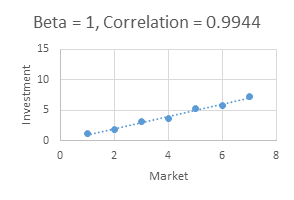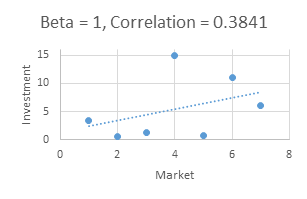The Beta for both examples above are 1, suggesting that each security very closely follows the market. However, in practice these two Betas are very different.

The graph on the left demonstrates that the investment and the market have historically moved in tandem, as the best fit line approaches each plotted point very closely (i.e., very high correlation). Thus, the Beta of 1 is meaningful and appears to be predictive of the future. The graph on the right demonstrates a relatively random relationship between the investment and the market (i.e., low correlation); by some coincidence, the "best fit line" happens to lead to a perfect Beta of 1, which of course is meaningless - there is no reason to expect the investment and the market to move in tandem in the future.

This illustration demonstrates one of the major drawbacks with Beta. If correlation is low, Beta is not useful and can even be misleading.

Another drawback of Beta is related to the traditional compliance disclosure that past results are no guarantee of future results - Beta is a formulaic calculation based on historical measurements, and thus not necessarily a great predictor of what is to come. Take a look at Chipotle's (CMG) 1 year rolling Beta below for a factual yet comical illustration of this - over a 5 year period, Beta ranged from 0 to 1.4.

This drawback is exacerbated by yet another, which is the need to decide the correct historical time period - how far back is "meaningful"? Chipotle's Beta on Yahoo Finance is .45, while Google lists Beta at .6 for Chipotle. Which one is right?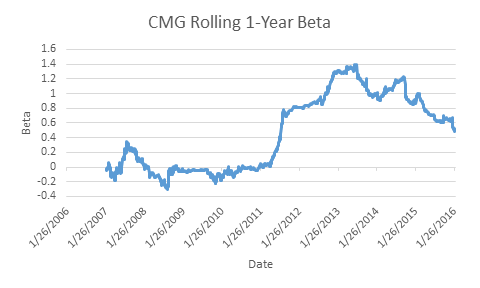These drawbacks collectively create a conundrum for investors looking to shape a portfolio by relying upon Beta.

Let's now discuss Delta. Delta is a lesser known term that is related to options. Like Beta, it is a measurement of the expected exposure to a referenced security. This is however where the similarities end. Beta, as mentioned, is a best fit line calculation between a given investment and a reference security; the reference security is typically the market (e.g., S&P 500 index), though it doesn't have to be.

Delta measures the expected exposure of an option to its reference security (e.g., the Delta of Apple (AAPL) options compared with Apple stock). It is calculated based on a few widely known variables, including the price of the reference security, the time to expiration of the option, and the implied volatility (future expected volatility as priced by the marketplace) of the option. Based on the way Delta is calculated, the value is current. Meaning that if the delta for an option is .8, then there is a very high likelihood that the option value will increase by approximately .8 for a \$1 movement in the referenced security.

For a call option, which provides the clients the right to purchase a stock, delta is bounded between 0 and 1 (i.e., if a security changes price, a call option following that security will change in the same direction somewhere between 0% and 100% - since a call option's Delta can't be negative, it won't change in the opposite direction, and since Delta can't exceed 1, it won't change more than the security did). For a put option, which provides the clients the right to sell a stock, delta is bounded between 0 and -1.

As a measurement, Delta cannot be used as broadly as Beta, since it can only be used with an option and the security the option follows. However, where it can be used, Delta is highly predictive of future relative exposure.

Let's look at Delta in practice.

A visual illustration of Call Delta vs. price of the reference security follows - y axis is delta, x axis is how far the reference stock is trading from the strike price. For this option, when strike = current market price (0% on the chart), Delta is about 0.5. As can be seen from the chart, Delta for an option changes as the reference security moves away from the strike price in either direction - Delta approaches 1 when the option is sufficiently in the money (e.g., stock is up about 30% vs. strike price), and Delta approaches 0 when the option is sufficiently out of the money (e.g., stock is down about 30% vs. strike). The implied volatility of the reference stock will affect how quickly Delta of an option changes as will the amount of time left to expiration - both conversations for another time.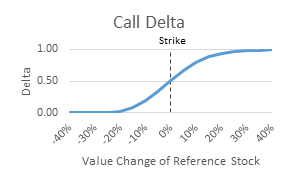Let's see how Delta works in action, starting with a simple example of a call option and then graduating to an options portfolio: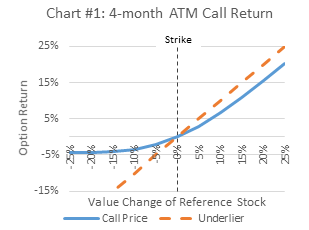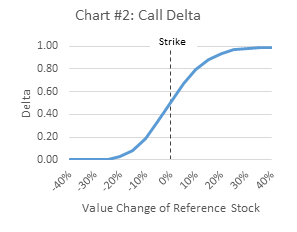Chart #1 is the classic shape of a call with time still left to maturity. If the reference security declines below the strike, the loss is limited to the price paid for the option while if the reference security increases the call participates with the gains. Chart #2 shows the previous graph of Delta based on the relative value of the referenced stock vs. strike price. Using the data in Chart #2, one can predict relatively accurately how much the price of the call will increase and decrease based on an increase or decrease in the price of the reference security.

Between the two charts, understanding ultimate exposure as well as relative exposure on a daily basis is very straightforward. For example, if the reference stock increased from 0% to 2%, the option should increase about 1% (Delta is about 0.5 for that range). However, if the reference stock increases from 30% to 32% (a 2% increase in total), the option value should increase about 2% (Delta is about 1 for that range).

Now that we have the basics, let's explore what happens when we combine options. Selling a "put spread" means we sell a put at a given strike and limit our downside by buying a put at a lower strike price - the lower the second strike price, the wider the spread, and the greater our risk (though the more premium we collect for taking that risk).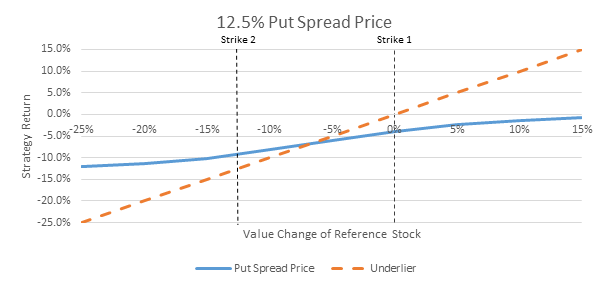Let's discuss the maximum exposure of the spread. If we covered ourselves 12.5% below where we sold the first put, our maximum exposure can at most be 12.5%. If the market declines -25% by expiration, then the put we sold would be worth -25% and the put we bought would be worth +12.5%, for a net loss of 12.5%. On the other hand, if the market finishes flat or positive, our value is 0, since both the put we sold and the put we bought are worth 0.

Can we figure out the Delta of the combined two option positions? Of course we can! A put spread is simply one long put and one short put so we can calculate the Delta of each and subtract. Below is a chart of the combined Deltas: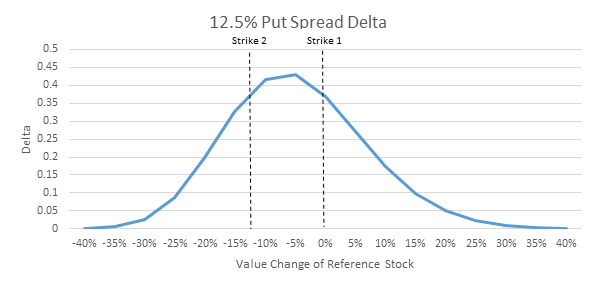Because we have two offsetting options, the Delta never goes to 1. Why? When the market is down significantly, the Delta for both puts is -1, so our long put and our short put result in a net Delta of -1 - (-1) = 0. This intuitively makes sense, because we know that once the market is down significantly more than -12.5%, it doesn't matter if it's down -30% or -40%, both puts are gaining value equally. If the market is up significantly, Delta for both puts is 0, bringing us back to a combined delta of 0.

The above chart shows the combined Deltas with 4 months to go prior to the options expiring. As we mentioned earlier, both time to expiration and volatility have an effect on delta - following is an example of how time affects Delta. The following chart is an illustration of the same 12.5% put spread with 2 weeks left to maturity. As can be seen, a large Delta exposure is concentrated around the center of the spread with almost no delta once either put is surpassed. At any given point in time, the overall Delta can be calculated to give an accurate expectation of the price movement relative to the reference security.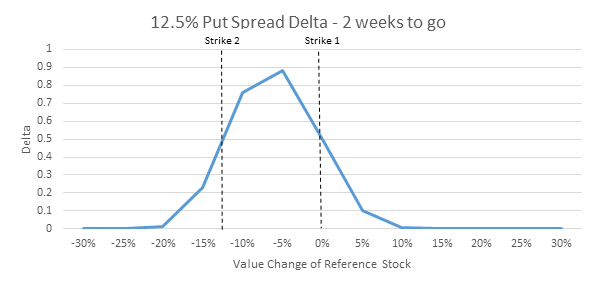Now that we all have a deeper understanding of Beta and Delta, I would like to bring this all back to crafting investment strategies. A large component of the investing public looks to Beta as a guide in making investment decisions. However, for the reasons we've mentioned, Beta can be an ineffectual tool in portfolio planning. A product with a low historical Beta may exhibit a future decline far greater than the market for any number of reasons.

As I've written about before, Funds and investment products often show a murky future, leaving a public averse to ambiguity unsatisfied with their investment options. So-called defensive strategies and tactical plays often seem to work for a period of time and abruptly stop working, leaving investors holding the bag (Contact me for many such examples).

In the hands of an experienced user, option strategies are uniquely able to provide a strong level of transparency along with tangible levels of risk and reward. There are a growing number of strategies in the market that look to take advantage of options. For example, our Exceed Investments products are engineered to take advantage of the unique qualities of options in providing a more defined and controlled exposure to the market. In the same way that Delta provides a more mathematically true approximation of the future than Beta does, defined outcome investing can offer a level of predictability unattainable in traditional equity investing.

 Strike is the value where the owner of a call has the contractual right to buy the stock and where the owner of the put would have the contractual right to sell the stock. For example, if Apple was trading \$200 and the strike of the call was \$195, the owner could exercise and buy at \$195. If the strike of the call was \$205, the owner would not exercise because they can simply buy it on the open market. (For reasons beyond the scope of this article, owners typically wait to expiration to exercise)

 Options are term based, with a specific maturity date. In that sense, they are similar to bonds. As such, there is a level of premium attributable to the value prior to maturity. Again a topic for another time.